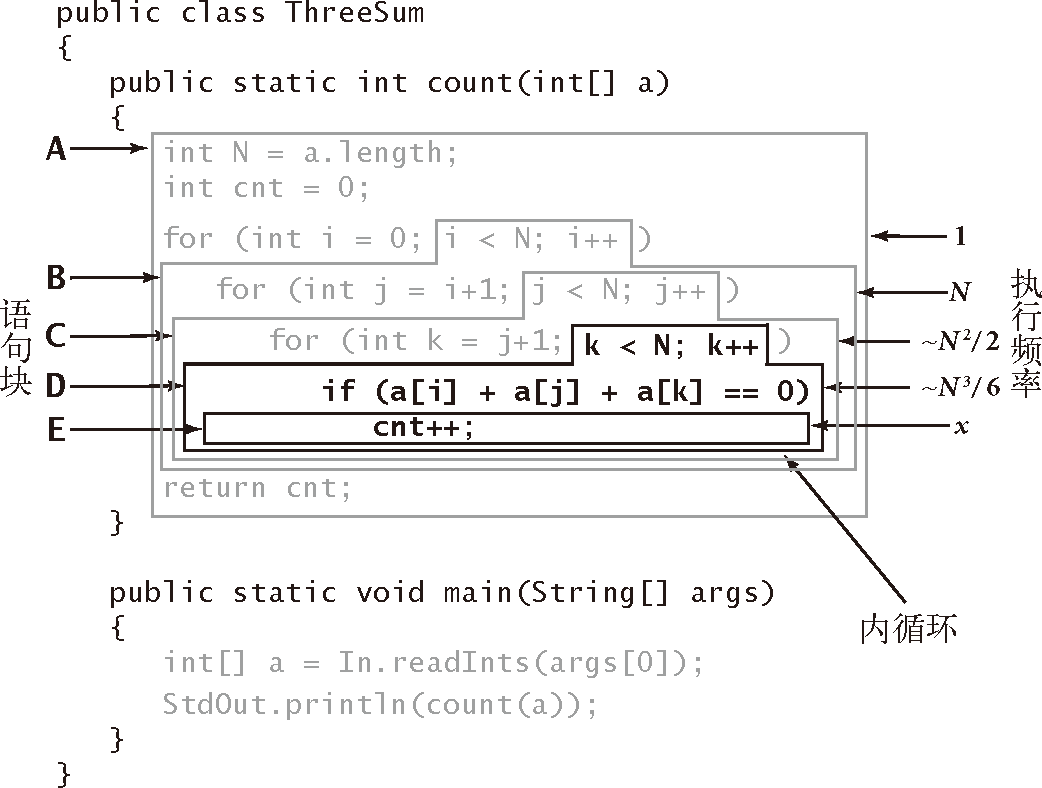# 算法（4th ed）(154)：基础——算法分析 6.3.3(数学模型：对增长数量级的猜想）E $t_0$ $x$(取决于输入) $t_0x$
D $t_1$ $N^3/6-N^2/2+N/3$ $t_1(N^3/6-N^2/2+N/3)$
C $t_2$ $N^2/2-N/2$ $t_2(N^2/2-N/2)$
B $t_3$ $N$ $t_3N$
A $t_4$ 1 $t_4$